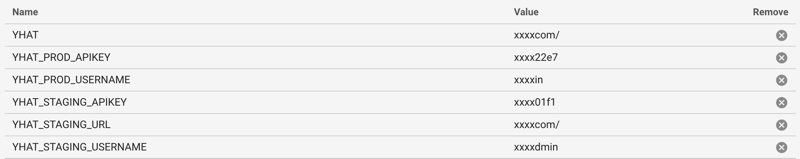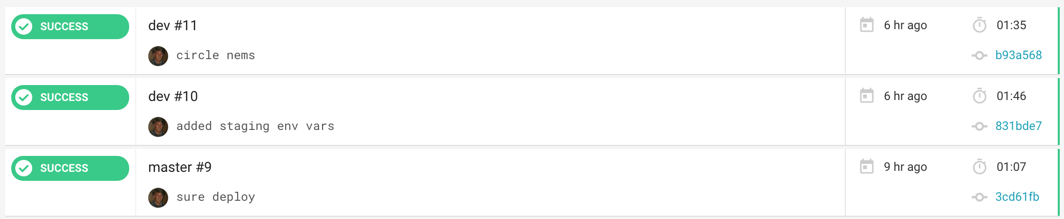# Deploying with CircleCi

Incorporating CircleCi into ScienceOps deployments is very straight forward.

In the example below we have a git repo with a simple model in it. Below is our file structure.

``````├── .git
├── circle.yml
└── model.py``````

A fairly common workflow for deploying follows the logic:

``````#some pseudo code:
if: code is pushed to the "master" branch:
build code on CircleCi
if: build succeeds and tests pass:
deploy to the production ScienceOps installation
else: dont deploy to ScienceOps
else if: code is pushed the "dev" branch:
deploy to a staging cluster``````

### Our `circle.yml` script:

``````machine:
timezone: America/New_York

# manually specify any dependencies
dependencies:
override:
- pip install scikit-learn pandas scipy yhat

# what will happen when we push to the master branch
deployment:
release:
branch: master
commands:
- >
YHAT_URL=\$YHAT
YHAT_APIKEY=\$YHAT_PROD_APIKEY
python model.py

# what will happen when we push to the dev branch
all:
branch: dev
commands:
- >
YHAT_URL=\$YHAT_STAGING_URL
YHAT_APIKEY=\$YHAT_STAGING_APIKEY
python model.py``````

### Our `model.py` script:

``````import os
import pandas as pd
from sklearn import linear_model
from sklearn import datasets

X = pd.DataFrame(iris.data[:,0:3], columns=iris.feature_names[0:3])
y = pd.DataFrame(iris.data[:,3:4], columns=iris.feature_names[3:4])

regr = linear_model.LinearRegression()
regr.fit(X, y)

from yhat import Yhat, YhatModel, preprocess, df_to_json

class LinReg(YhatModel):
REQUIREMENTS=["pandas","scikit-learn"]
@preprocess(in_type=pd.DataFrame, out_type=dict)
def execute(self, data):
prediction = regr.predict(pd.DataFrame(data)).tolist()
return {"prediction":prediction}

if __name__ == '__main__':
yh = Yhat(
os.environ['YHAT_APIKEY'],
os.environ['YHAT_URL'],
)

yh.deploy("LinearRegression", LinReg, globals(), sure=True, autodetect=False)``````

In CircleCi, all we need to do is add our Environment Variables for the Production and Staging Username, APIKEY, and URLFrom here, all we need to do is run:

`\$ git push origin master`

and we should see 1. Code pushed to github 2. A build begin on CircleCi 3. A new model version deployed to ScienceOps